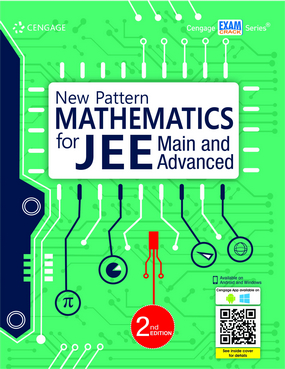###### Home
>

Test Prep### New Pattern Mathematics for JEE Main and Advanced, 2E

Author(s): Cengage India

ISBN: 9789353502102

Edition: 2nd

#### Rs. 825

Binding: Paperback

Pages: 1180

Trim Size : 279 x 216 mm

New Pattern Mathematics for JEE Main and Advanced (2e) is a vast bank of questions designed on the latest trends appearing in JEE Main and Advanced. It offers the aspirants an opportunity to familiarize themselves with the nature and level of complexity of questions asked in JEE Main and Advanced.

The content in the book has been divided into five broad sections: Algebra, Trigonometry, Coordinate Geometry, Calculus, and Vectors and 3D Geometry. Each section in turn has been sub-divided into chapters comprising all types of questions asked in JEE Main and Advanced. Solutions given at the end of the book help aspirants in recognizing the methodology by which tricky questions can be cracked in the examination, thus enhancing their problem-solving ability. The Cengage App connected with the book features chapterwise mini tests to practice for JEE Main and Advanced.

• Reflects contemporary trends in the JEE
• Includes all types of questions asked in the examination
• Matching Column Type
• Hints and Solutions provided at the book end
• Book updated with solutions of JEE Advanced 2018 and 2019 papers
• FREE mini practice tests in the App (Windows/Android) with Solutions and Analysis

Algebra

Chapter 1: Inequalities and Absolute Value

Chapter 2: Theory of Equations

Chapter 3: Complex Numbers

Chapter 4: Progression and Series

Chapter 5: Inequalities Involving Means

Chapter 6: Permutation and Combination

Chapter 7: Binomial Theorem

Chapter 8: Determinants

Chapter 9: Matrices

Chapter 10: Probability

Trigonometry

Chapter 11: Logarithm and Its Applications

Chapter 12: Trigonometric Functions

Chapter 13: Trigonometric Ratios for Compound, Multiple, Sub-multiple Angles, and Transformation Formulas

Chapter 14: Trigonometric Equations

Chapter 15: Inverse Trigonometric Functions

Chapter 16: Solutions and Properties of Triangle

Coordinate Geometry

Chapter 17: Coordinate System

Chapter 18: Straight Lines

Chapter 19: Pair of Straight Lines

Chapter 20: Circle

Chapter 21: Parabola

Chapter 22: Ellipse

Chapter 23: Hyperbola

Calculus

Chapter 24: Functions

Chapter 25: Limits

Chapter 26: Continuity and Differentiability

Chapter 27: Methods of Differentiation

Chapter 28: Application of Derivatives

Chapter 29: Monotonicity and Maxima–Minima of Functions

Chapter 30: Indefinite Integration

Chapter 31: Definite Integration

Chapter 32: Area

Chapter 33: Differential Equations

Vectors and 3D-Geometry

Chapter 34: Introduction to Vectors

Chapter 35: Different Products of Vectors and Their Geometrical Applications

Chapter 36: Three-Dimensional Geometry

Hints and Solutions

Appendix

Solved JEE Advanced 2018 and 2019 Papers